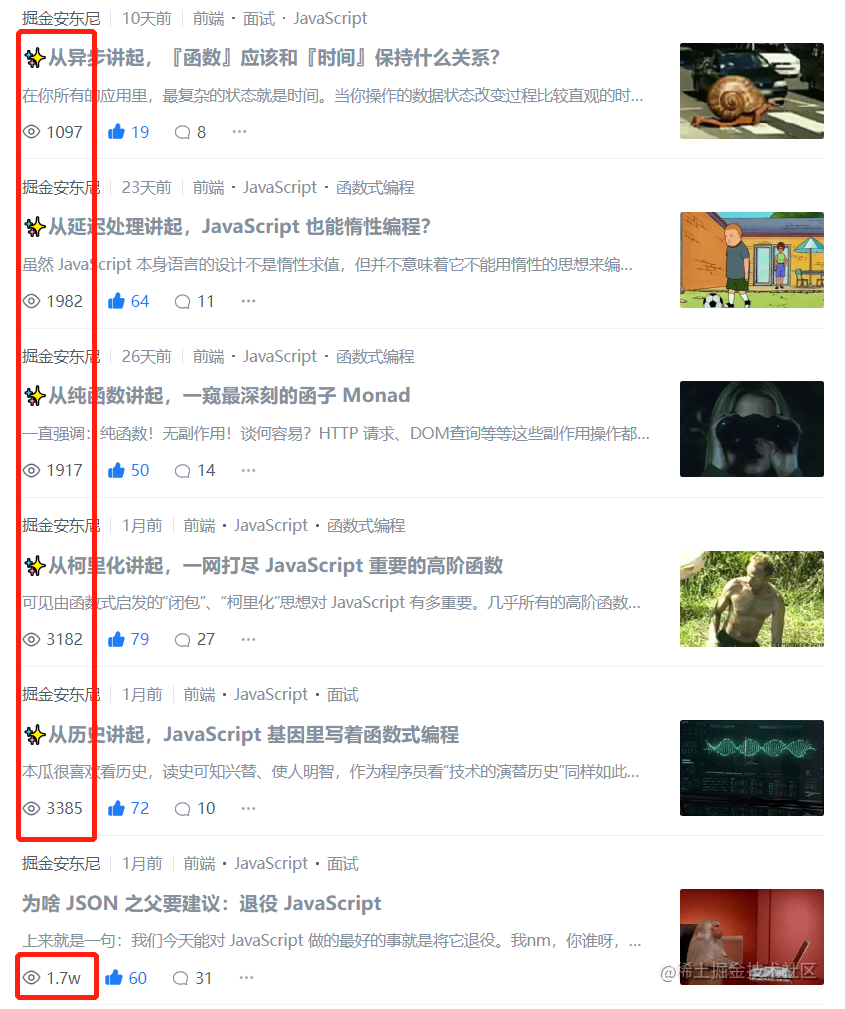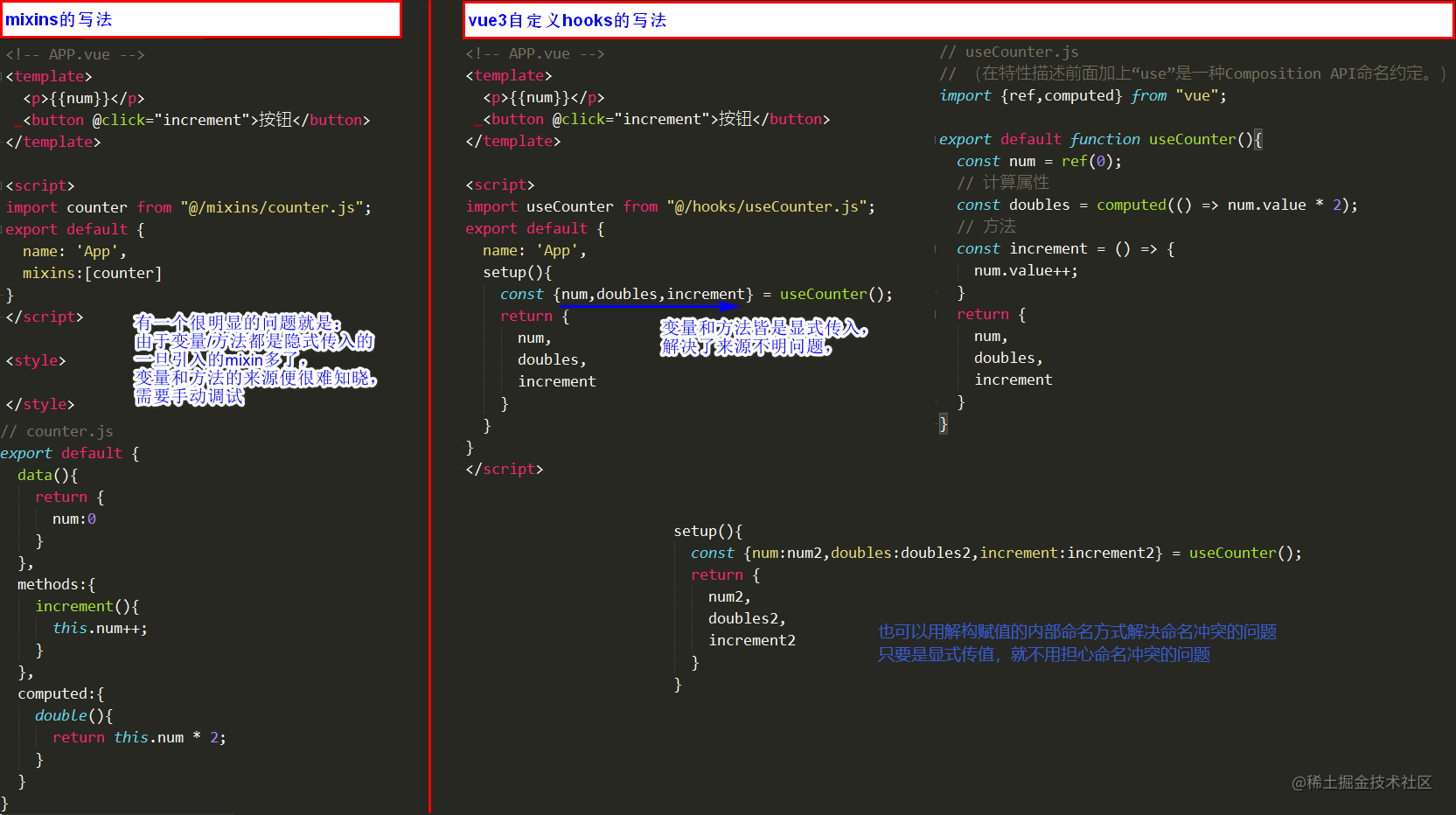# ✨从代码复用讲起，专栏阶段性作结，聊聊？## 回顾

### 数据一览1. 平台对新文章的推送策略从 9 月份之后发生变化，转变为更侧重于推送旧的好文章；
2. 专栏内容相对较干，更多人来社区看文章或图一乐、或为解决问题、或为面试、或为收集好资源；
3. 更文频率下降，导致账号整体流量偏小（因为同时段在关注另一件事）；### 不忘初心

1. 关注 JavaScript 两个核心 —— “闭包” 和 “异步”；
2. 函数式编程真的串联了这两个核心吗？
3. 从高阶函数到函数组合；
4. 从无副作用到延迟处理；
5. 从函数响应式到事件流；
6. 谈代码重用；

1. 闭包的起源，闭包刻在 javaScript 基因里；
2. 柯里化思想，一网打尽高阶函数；
3. 纯函数、无副作用、函数组合、函数怎样“尽可能保持纯”；
4. 延迟处理、JS 惰性编程，联系闭包和异步；
5. 函数响应式编程 FRP, RxJS Observable 事件流及实战；
6. 本篇后文将浅谈代码重用；

OK，方向好像确实是这么一个方向，没走偏。

fine，理论指导实践，实践是检验真理的标准。所以这里是“阶段性”作结，

## 代码复用

### Vue2 mixin

1. 如果是data函数的返回值对象 返回值对象默认情况下会进行合并； 如果data返回值对象的属性发生了冲突，那么会保留组件自身的数据；

2. 如果是生命周期钩子函数 生命周期的钩子函数会被合并到数组中，都会被调用； mixin中的生命周期钩子函数会比组件中的生命周期钩子函数先执行(全局mixin先于局部mixin,局部mixin先于组件)；

3. 值为对象的选项，例如 methods、components 和 directives，将被合并为同一个对象。 比如都有methods选项，并且都定义了方法，那么它们都会生效； 但是如果对象的key相同，那么会取组件对象的键值对；

mixin 缺点：

1. 变量来源不明确（隐式传入），不利于阅读，使代码变得难以维护。

1. 多个mixins的生命周期会融合到一起运行，但是同名属性、同名方法无法融合，可能会导致冲突、很容易制造混乱。

2. mixins和组件可能出现多对多的关系，复杂度较高（即一个组件可以引用多个mixins，一个mixins也可以被多个组件引用）。``````情况 1. 默认绑定

this 的绑定其实也是为了代码重用，同样搞得人头疼。完全不符合 JS 轻量、简单的气质。

### Vue3 Setup### React class

React 也是啊。React V16.8 hooks 出来之前的 class 组件，this 的绑定之麻烦，定位问题查询起来之麻烦，也是 this 的指向规则、以及隐式的输入、输出导致的。

``````this.props.xxx();
this.props.aaa();
this.props.bbb();

### React hooks

``````const { xxx } = useXXX();
const { aaa } = useAAA();
const { bbb } = useBBB();

class Component：

``````class ExampleOfClass extends Component {
constructor(props) {
super(props)
this.state = {
count: 1
}
}
handleClick = () => {
let { count } = this.state
this.setState({
count: count+1
})
}
render() {
const { count } = this.state
return (
<div>
<p>you click { count }</p>
<button onClick={this.handleClick}>点击</button>
</div>
)
}
}

hooks：

### 小结

1. 我们确实不喜欢隐式的输入、输出，对于代码的可读性太不又好了；
2. 我们在复用的时候讨厌 this 指来指去；
3. 千万不要在查找属性的时候，又要查同级的组件、父组件、父父组件，从哪来、到哪去，一定给说明白了。

## 复用思考

react 相对于 vue2 本身就是比较偏“函数式”的。

## 专栏总结

OK，以上便是本篇分享，专栏第 7 篇，希望各位工友喜欢~ 欢迎点赞、收藏、评论 🤟

## 彩蛋翻译（写的很好！！关于“用 JS 代码解释 JS Monad 如何理解”~）

``````var sine = function(x) { return Math.sin(x) };

``````var cube = function(x) { return x * x * x };

``````var sineCubed = cube(sine(x))

``````var compose = function(f, g) {
return function(x) {
return f(g(x));
};
};

var sineOfCube = compose(sine, cube);
var y = sineOfCube(x);

``````var cube = function(x) {
console.log('cube was called.');
return x * x * x;
};

``````var sine = function(x) {
return [Math.sin(x), 'sine was called.'];
};

var cube = function(x) {
return [x * x * x, 'cube was called.'];
};

``````cube(3) // -> [27, 'cube was called.']

compose(sine, cube)(3) // -> [NaN, 'sine was called.']

sin 函数要计算一个数组的正弦，这显然不能得出正确的值

``````var composeDebuggable = function(f, g) {
return function(x) {
var gx = g(x),      // e.g. cube(3) -> [27, 'cube was called.']
y  = gx,     //                 27
s  = gx,     //                 'cube was called.'
fy = f(y),      //     sine(27) -> [0.956, 'sine was called.']
z  = fy,     //                 0.956
t  = fy;     //                 'sine was called.'

return [z, s + t];
};
};

composeDebuggable(sine, cube)(3)
// -> [0.956, 'cube was called.sine was called.']

cube 接受一个 number ，返回一个 number 和 string 的元组；

``````// 写法 1
cube :: Number -> (Number,String)

``````// 写法 2
cube :: (Number,String) -> (Number,String)

``````var bind = function(f) {
return function(tuple) {
var x  = tuple,
s  = tuple,
fx = f(x),
y  = fx,
t  = fx;

return [y, s + t];
};
};

``````var f = compose(bind(sine), bind(cube));
f([3, '']) // -> [0.956, 'cube was called.sine was called.']

``````// unit :: Number -> (Number,String)
var unit = function(x) { return [x, ''] };

``````// unit :: Number -> (Number,String)
var unit = function(x) { return [x, ''] };

var f = compose(bind(sine), bind(cube));
f(unit(3)) // -> [0.956, 'cube was called.sine was called.']

// or ...
compose(f, unit)(3) // -> [0.956, 'cube was called.sine was called.']

unit 函数不仅能对输入的参数进行改造，还能对 return 输出的函数的类型进行改造：

``````// round :: Number -> Number
var round = function(x) { return Math.round(x) };

// roundDebug :: Number -> (Number,String)
var roundDebug = function(x) { return unit(round(x)) };

``````// lift :: (Number -> Number) -> (Number -> (Number,String))
var lift = function(f) {
return function(x) {
return unit(f(x));
};
};

// or, more simply:
var lift = function(f) { return compose(unit, f) };

``````var round = function(x) { return Math.round(x) };

var roundDebug = lift(round);

var f = compose(bind(roundDebug), bind(sine));
f(unit(27)) // -> [1, 'sine was called.']

bind ：可以将可调式的函数转换成可组合的形式；

Number -> (Number,String) 改造成 (Number,String) -> (Number,String)

unit ： 可以将简单的值放入容器，将其转换成可调试的格式；

Number -> (Number,String)

lift ： 可以将简单函数转换为可调试的函数；

(Number -> Number) 改造成 (Number -> (Number,String))

``````// children :: HTMLElement -> [HTMLElement]
var children = function(node) {
var children = node.childNodes, ary = [];
for (var i = 0, n = children.length; i < n; i++) {
ary[i] = children[i];
}
return ary;
};

// e.g.
// -> [
//      "Translation from Haskell to JavaScript...",
//      <span class=​"edit">​…​</span>​
//    ]

``````var grandchildren = compose(children, children)

``````// grandchildren :: HTMLElement -> [HTMLElement]
var grandchildren = function(node) {
var output = [], childs = children(node);
for (var i = 0, n = childs.length; i < n; i++) {
output = output.concat(children(childs[i]));
}
return output;
};

``````// unit :: a -> [a]
var unit = function(x) { return [x] };

// bind :: (a -> [a]) -> ([a] -> [a])
var bind = function(f) {
return function(list) {
var output = [];
for (var i = 0, n = list.length; i < n; i++) {
output = output.concat(f(list[i]));
}
return output;
};
};

``````var div = document.getElementsByTagName('div');
var grandchildren = compose(bind(children), bind(children));

grandchildren(unit(div))
// -> [<h1>…</h1>, <p>…</p>, ...]OK，以上便是本篇分享，希望各位工友喜欢~ 欢迎点赞、收藏、评论 🤟

😹 加我微信 ATAR53，拉你入群，定期抽奖、粉丝福利多多。只学习交友、不推文卖课~

😸 我的公众号：掘金安东尼，在上面，不止编程，更多还有生活感悟~

😺 我的 GithubPage: tuaran.github.io，它已经被维护 4 年+ 啦~